## Tetrahedron Inscribing

Pick four points at random on the surface of a unit Sphere. Find the distribution of possible volumes of (nonregular) Tetrahedra. Without loss of generality, the first point can be chosen as (1, 0, 0). Designate the other points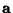,, and. Then the distances from the first Vertex are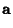(1)(2)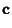(3)

The average volume is then(4)

where(5)

and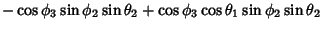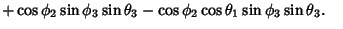(6)
The integrals are difficult to compute analytically, but 107 computer Trials give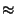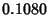(7)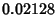(8)(9)

See also Point-Point Distance--1-D, Triangle Inscribing in a Circle, Triangle Inscribing in an Ellipse

References

Buchta, C. A Note on the Volume of a Random Polytope in a Tetrahedron.'' Ill. J. Math. 30, 653-659, 1986.